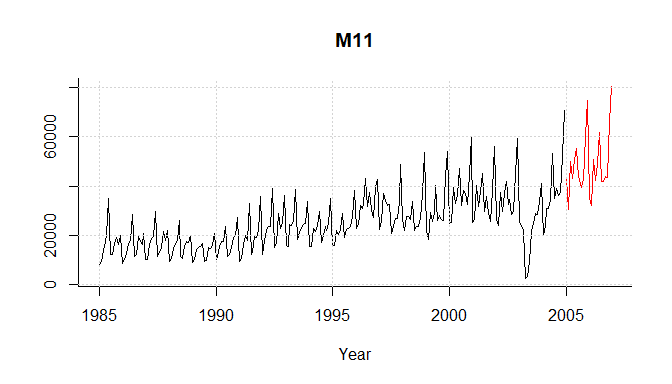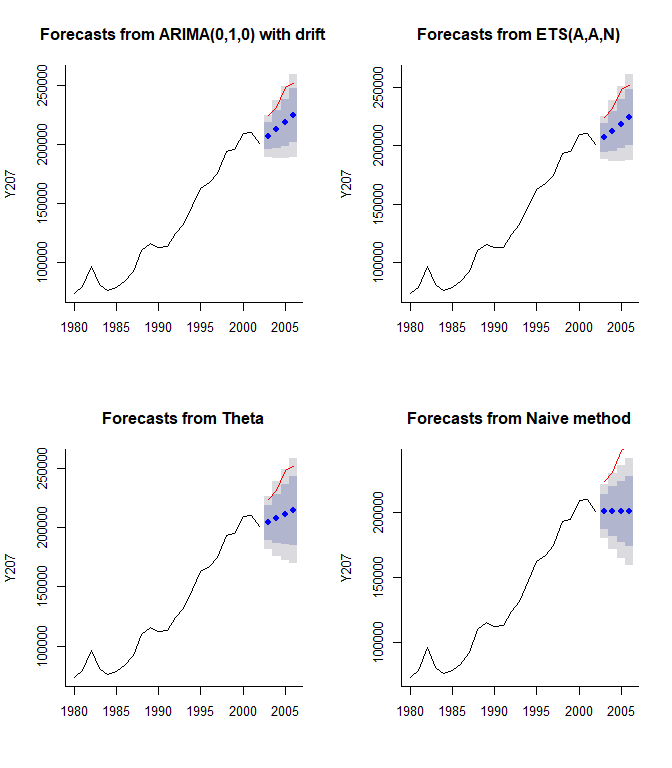# Analysis with the 2010 tourism forecasting competition data

## Tourism data

This vignette introduces the Tcomp R package, which is a convenient wrapper around the univariate versions of the data used in the Tourism Forecasting competition described in Athanasopoulos et al (2011), originally in the International Journal of Forecasting (2011) 27(3), 822-844, available in full from Hyndman’s blog. Unfortunately, the explanatory variables are not available, so only univariate forecasting methods can be tested with this data collection. The data available here are the same as those used in the Kaggle competition.

The original data are also available from Hyndman’s site, in CSV format, and have been wrapped into the Tcomp package with his permission. Any errors that occurred in the wrapping process are the package author’s responsibility and if there is doubt, the R version should be checked against the CSV originals. However, testing has been pretty thorough, and further down in this vignette we successfully reproduce exactly the mean absolute percentage error of the naive forecasts that are inter alia reported in that 2011 article. Please report errors as issues on this package’s GitHub page.

## The data

The data are all in a lazy-loaded list called tourism which consists of 1311 objects. tourism is a list of class Mcomp, a class inherited from Hyndman et al.’s Mcomp package which has the data from the M1 and M3 forecasting competitions.

length(tourism)
 1311
class(tourism)
 "Mcomp"

Each of the 1311 objects in tourism is a list of class Mdata and it has the same elements as the data in the Mcomp package. The example here is one of the annual time series:

tourism$Y5 Series: Y5 Type of series: TOURISM Period of series: YEARLY Series description: No description available HISTORICAL data Time Series: Start = 1973 End = 2003 Frequency = 1  27186 27921 32385 38746 41356 51851 58426 61869 62926 58170  62941 71411 79687 90608 109203 123737 118919 118556 136967 172200  219706 236384 249266 275892 235006 170716 172166 193837 173359 172654  116966 FUTURE data Time Series: Start = 2004 End = 2007 Frequency = 1  169661 204625 213837 234763 Here is the full structure of each Mdata object, again using the fifth yearly series as an example: str(tourism$Y5)
List of 9
$st : chr "Y5"$ period     : chr "YEARLY"
$x : Time-Series [1:31] from 1973 to 2003: 27186 27921 32385 38746 41356 ...$ xx         : Time-Series [1:4] from 2004 to 2007: 169661 204625 213837 234763
$h : num 4$ n          : num 31
$sn : chr "Y5"$ type       : chr "TOURISM"
$description: chr "No description available" - attr(*, "class")= chr "Mdata" The Mcomp package also provides a convenient plotting method for Mdata objects, combining the training and testing periods of the data. Shown here with one of the monthly time series: par(bty = "l") plot(tourism$M11); grid()## Summary information

Some of the descriptive statistics from Table 3 of Athanasopoulos et al. are reproduced below.

library(dplyr, warn.conflicts = FALSE)
library(xtable)
lengthsm <- sapply(subset(tourism, "monthly"), function(s){length(s$x) + length(s$xx)})
lengthsq <- sapply(subset(tourism, "quarterly"), function(s){length(s$x) + length(s$xx)})
lengthsy <- sapply(subset(tourism, "yearly"), function(s){length(s$x) + length(s$xx)})

tmp <- data.frame(series_length = c(lengthsm, lengthsq, lengthsy),
series_type = c(rep("Monthly", length(lengthsm)),
rep("Quarterly", length(lengthsq)),
rep("Yearly", length(lengthsy))
)) %>%
group_by(series_type) %>%
summarise(
TotalNumber = length(series_length),
MeanLength = round(mean(series_length), 1),
MedianLength = median(series_length),
MinLength = min(series_length),
MaxLength = max(series_length)
) %>%
select(-series_type) %>%
t() %>%
as.matrix()
colnames(tmp) <- c("Monthly", "Quarterly", "Yearly")
xtable(tmp, digits = 1)
Monthly Quarterly Yearly
TotalNumber 366.0 427.0 518.0
MeanLength 298.6 99.6 24.5
MedianLength 330.0 110.0 27.0
MinLength 91.0 30.0 11.0
MaxLength 333.0 130.0 47.0

Comparison with the original shows that while the number of series, median length, minimum length and maximum length match exactly; mean length only matches if the results above are truncated rather than rounded (ie 298.6 becomes 298). Given the other statistics match, and the mean absolute percentage error of naive forecasts match (see below), this discrepancy is assumed to be a minor rounding error in Athanasopoulos et al.

## Analysis

The Tcomp package provides forecast_comp(), a wrapper around functions from Hyndman’s forecast package which performs forecasts based on:

• exponential smoothing state space
• ARIMA
• theta
• naive or seasonal naive

methods and returns mean absolute percentage error (MAPE) and mean absolute scaled error (MASE) for the forecasts compared to the test set, at given testing horizons

forecast_comp(tourism[], tests = list(6, 12, 24))
               6         12          24
ARIMA 25.9180306 11.1240673  1.76714721
ETS    4.9116432  9.0028074 16.91546830
Theta  7.9096803  4.6594952 11.86635472
Naive 16.3879599  4.7619048  4.34782609
ARIMA  1.6232449  0.4403888  0.07662208
ETS    0.3076159  0.3564106  0.73344106
Theta  0.4953828  0.1844640  0.51451557
Naive  1.0263770  0.1885182  0.18851823

The first four rows are MAPE and the last four are MASE; note that this is not possible to tell from the output without knowing in advance.

For this particular series (which is a monthly one), taking the MASE (which I think is the better measure), only the ARIMA model out-performed the seasonal naive model (which is forecasts values as being the samne as the most recent observed value for that month).

forecast_comp() comes with an optional plot function which compares the four forecasting methods to actuals:

forecast_comp(tourism[], tests = list(1,2,3,4), plot = TRUE)1         2         3         4
ARIMA  7.882166  8.206717 12.078049 11.002449
ETS    7.881325  8.205743 12.076994 11.001263
Theta  8.951896 10.280864 14.978957 14.816260
Naive 10.473181 13.229221 19.101916 20.236285
ARIMA  2.012628  2.162056  3.412948  3.153226
ETS    2.012413  2.161800  3.412649  3.152886
Theta  2.285772  2.708490  4.232670  4.246237
Naive  2.674216  3.485233  5.397712  5.799579

In this case, we see that actual growth exceeded the 80% prediction interval for each method (but not always the 95% prediction interval).

## Reproducing some of the competition results

forecast_comp() is designed to be used in a larger scale than one series at a time. Here is a more extended use, aimed at re-creating some of the results reported in Athanasoupolus et al.

library(Tcomp)
library(dplyr)
library(tidyr)
library(parallel)

# this function runs the four standard models in forecast_comp
# on a large chunk of the competition series from either Mcomp or Tcomp.
# The aim is to help comparisons with Athanasopoulos et al.
#
# The use of makePSOCKcluster and parLapply speeds up the analysis nearly four fold on my laptop
# eg running the test on all the yearly tourism series takes 12 seconds rather than 44 seconds.

#' @param dataobj a list of class Mcomp such as M3 or tourism
#' @param cond1 a condition for subsetting dataobj eg "yearly"
#' @param tests a list of different horizons at which to return the MASE for four different models
#'
#' @return a data.frame with \code{length(tests) + 2} columns and 8 rows
accuracy_measures <- function(dataobj, cond1, tests){
cores <- detectCores()

cluster <- makePSOCKcluster(max(1, cores - 1))

clusterEvalQ(cluster, {
library(Tcomp)
library(forecast)
})

results <- parLapply(cluster,
subset(dataobj, cond1),
forecast_comp,
tests = tests)

results_mat <- do.call(rbind, results)
nr <- nrow(results_mat)

tmp <- as.data.frame(results_mat) %>%
mutate(measure = rep(rep(c("MAPE", "MASE"), times = c(4, 4)), times = nr / 8)) %>%
mutate(method = rownames(results_mat)) %>%
gather(horizon, mase, -method, -measure) %>%
group_by(method, measure, horizon) %>%
summarise(result = round(mean(mase), 2)) %>%
ungroup() %>%
mutate(horizon = factor(horizon, levels = colnames(results[]))) %>%
arrange(measure) %>%
as.data.frame()

stopCluster(cluster)

return(tmp)
}

accuracy_measures(tourism, "monthly", list(1, 2, 3, 6, 12, 18, 24, 1:3, 1:12, 1:24))
accuracy_measures(tourism, "quarterly", list(1, 2, 3, 4, 6, 8, 1:4, 1:8))
accuracy_measures(tourism, "yearly", list(1, 2, 3, 4, 1:2, 1:4))

The code above isn’t run in the build of the vignette for reasons of time (takes a few minutes to run on my laptop, making it to intensive for a CRAN build). The results are available in the package however as a list called Tcomp_reproduction

xtable(Tcomp_reproduction$monthly) method measure 1 2 3 6 12 18 24 1-3 1-12 1-24 1 ARIMA MAPE 18.74 21.04 21.21 23.38 26.75 24.43 35.41 20.33 22.70 26.13 2 ETS MAPE 18.07 17.54 18.15 20.55 20.65 18.89 23.51 17.92 19.64 21.06 3 Naive MAPE 19.89 21.56 20.64 20.94 21.09 19.97 22.30 20.70 21.38 22.56 4 Theta MAPE 19.52 20.39 20.67 20.63 21.58 18.77 23.11 20.19 21.33 22.39 5 ARIMA MASE 1.08 1.35 1.48 1.56 1.23 2.09 1.68 1.31 1.38 1.60 6 ETS MASE 1.25 1.35 1.39 1.41 1.20 1.87 1.64 1.33 1.34 1.54 7 Naive MASE 1.29 1.50 1.47 1.55 1.16 1.89 1.57 1.42 1.45 1.63 8 Theta MASE 1.44 1.60 1.83 1.52 1.22 1.84 1.57 1.62 1.50 1.65 xtable(Tcomp_reproduction$quarterly)
method measure 1 2 3 4 6 8 1-4 1-8
1 ARIMA MAPE 13.35 13.02 15.06 14.78 16.52 23.08 14.05 16.47
2 ETS MAPE 11.59 12.30 13.54 14.43 14.83 22.03 12.97 15.31
3 Naive MAPE 13.95 14.79 14.41 13.61 18.02 21.15 14.19 16.46
4 Theta MAPE 13.75 13.81 14.39 14.65 15.02 21.31 14.15 15.93
5 ARIMA MASE 1.20 1.40 1.31 1.35 1.97 1.99 1.31 1.60
6 ETS MASE 1.24 1.39 1.20 1.36 1.92 1.99 1.30 1.59
7 Naive MASE 1.41 1.53 1.30 1.27 2.22 1.93 1.38 1.70
8 Theta MASE 1.58 1.53 1.30 1.34 1.85 1.91 1.44 1.66
xtable(Tcomp_reproduction\$yearly)
method measure 1 2 3 4 1-2 1-4
1 ARIMA MAPE 26.28 26.60 32.42 37.20 26.44 30.62
2 ETS MAPE 23.02 21.81 25.33 29.53 22.41 24.92
3 Naive MAPE 21.47 20.80 24.12 28.05 21.14 23.61
4 Theta MAPE 22.70 20.95 22.99 26.66 21.83 23.33
5 ARIMA MASE 1.82 2.67 3.71 4.57 2.25 3.19
6 ETS MASE 1.64 2.53 3.49 4.35 2.09 3.00
7 Naive MASE 1.58 2.51 3.54 4.40 2.05 3.01
8 Theta MASE 1.56 2.34 3.15 3.87 1.95 2.73

Comparing the results above to Tables 4, 5 and 6 in Athanasopoulos et al. we get exact matches for the mean absolute percentage error (MAPE) of the Naive forecasts, but not for any other rows. It is expected that the methods for ARIMA, ETS and Theta forecasts in the current forecast package differ slightly from the methods used in the 2011 article. However, I don’t have an explanation for why the mean absolute scaled error estimates above for the naive forecasts don’t match those in the 2011 article. The numbers I obtain are slightly highter than the published numbers; obviously there is a small difference in how MASE is being calculated (my code above uses forecast::accuracy() with the default values). I’m confident the data is identical because of the exact match of the MAPE values of all three sets of naive forecasts (there is less discretion in exactly how to measure MAPE).